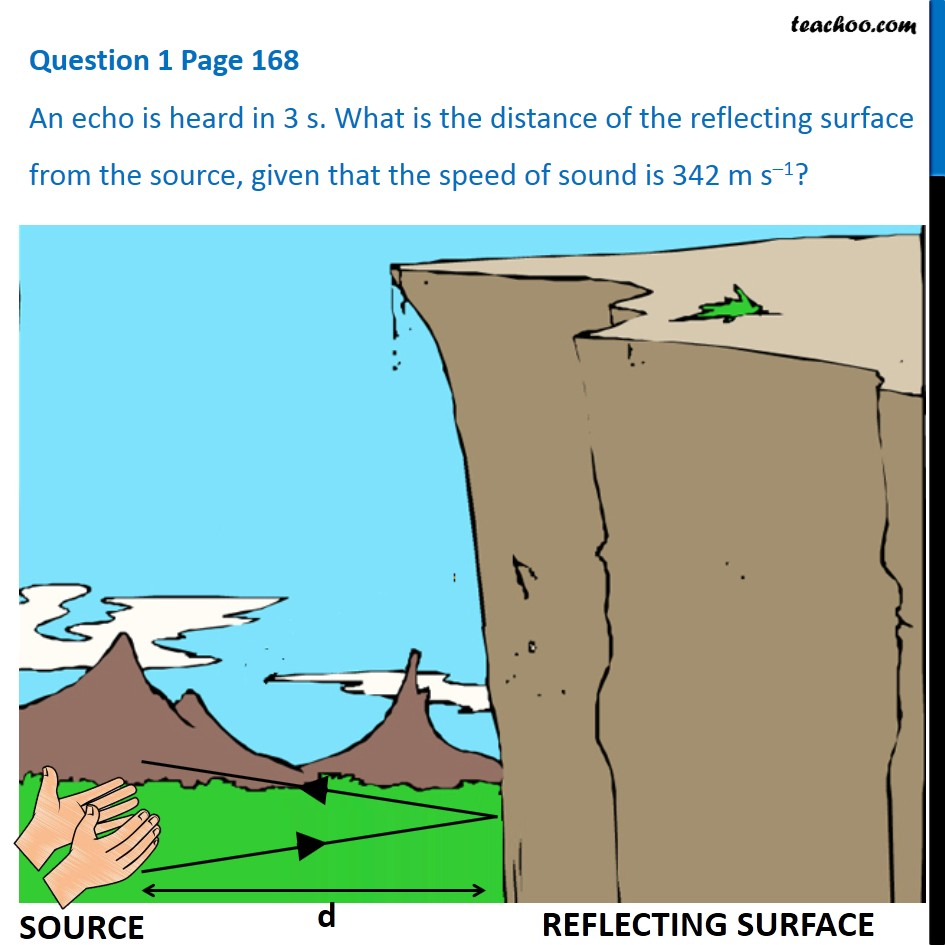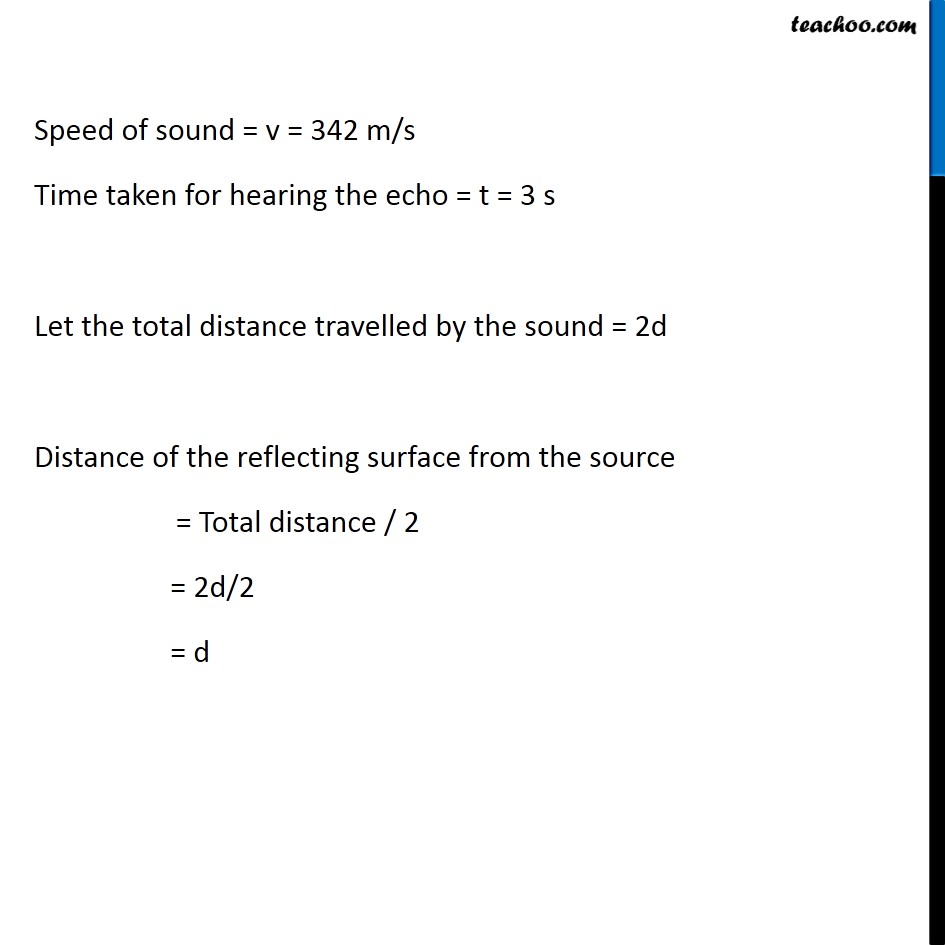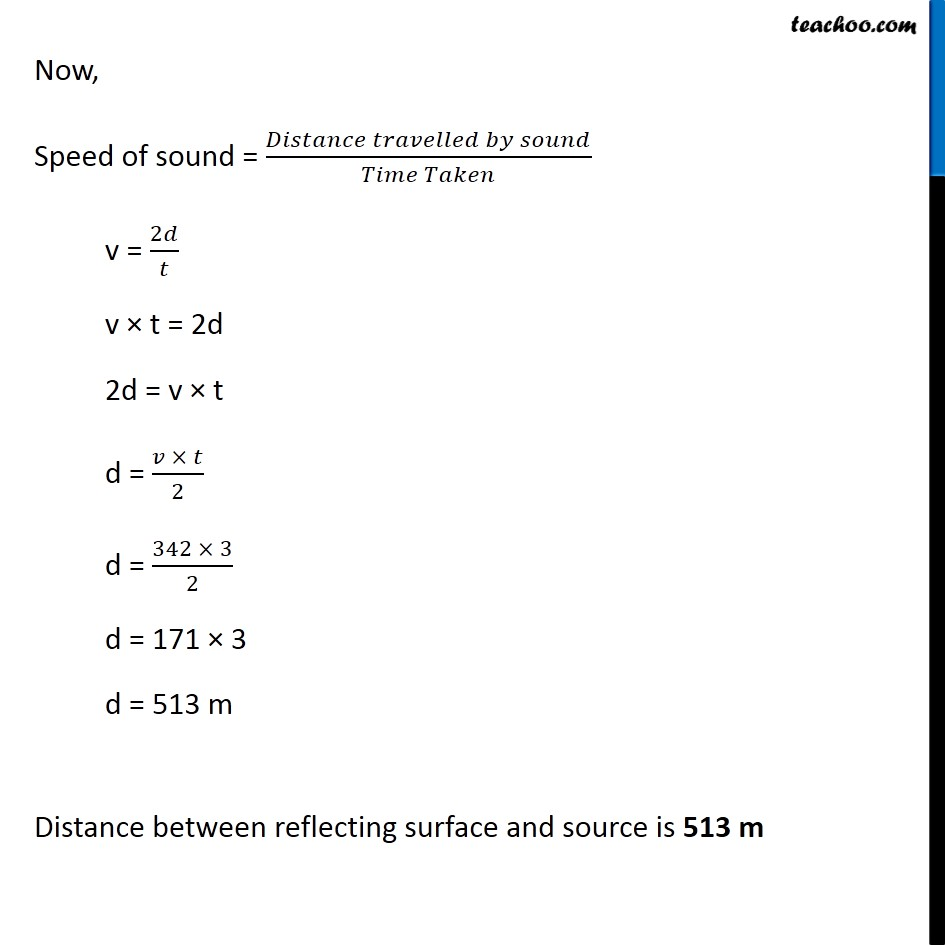Questions from inside the book

Class 9
Chapter 12 Class 9 - SoundLearn in your speed, with individual attention - Teachoo Maths 1-on-1 Class

### Transcript

Question 1 Page 168 An echo is heard in 3 s. What is the distance of the reflecting surface from the source, given that the speed of sound is 342 m s–1? Speed of sound = v = 342 m/s Time taken for hearing the echo = t = 3 s Let the total distance travelled by the sound = 2d Distance of the reflecting surface from the source = Total distance / 2 = 2d/2 = d Now, Speed of sound = (𝐷𝑖𝑠𝑡𝑎𝑛𝑐𝑒 𝑡𝑟𝑎𝑣𝑒𝑙𝑙𝑒𝑑 𝑏𝑦 𝑠𝑜𝑢𝑛𝑑)/(𝑇𝑖𝑚𝑒 𝑇𝑎𝑘𝑒𝑛) v = 2𝑑/𝑡 v × t = 2d 2d = v × t d = (𝑣 × 𝑡)/2 d = (342 × 3)/2 d = 171 × 3 d = 513 m Distance between reflecting surface and source is 513 m